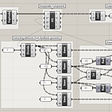# Articulation Points and Bridges

a) Cut vertex v and its incident edges.

b) Run O(V+E) DFS(or BFS) and see if the number of CCs increases.

c) If yes, v is an articulation point. Restore v and its incident edges.

# Leetcode Problem

## Naive TLE solution

1. block each connect to see whether all nodes still can be connected after block.
2. If can, then it is not a critical connection. If not, it is.
`class Solution:    def criticalConnections(self, n: int, connections: List[List[int]]) -> List[List[int]]:        def critical(k):            uf = {}            def find(x):                uf.setdefault(x,x)                if uf[x]!=x:                    uf[x]=find(uf[x])                return uf[x]            def union(x,y):                uf[find(y)]=find(x)            for i, (u,v) in enumerate(connections):                if i!=k:union(u,v)            return len({find(i) for i in range(n)})!=1        return [connection for k,connection in enumerate(connections) if critical(k)]`

## Tarjan similar solution

`class Solution:    def criticalConnections(self, n: int, connections: List[List[int]]) -> List[List[int]]:        graph = collections.defaultdict(list)        for u, v in connections:            graph[u].append(v)            graph[v].append(u)        connections = {tuple(sorted(connection)) for connection in connections}        ranks = [-2]*n                def dfs(node, depth):            if ranks[node] >=0:return ranks[node]            ranks[node] = depth            min_back_depth = n            for neighbor in graph[node]:                if ranks[neighbor] == depth-1:continue                back_depth = dfs(neighbor, depth+1)                if back_depth <= depth:                    connections.discard(tuple(sorted([node, neighbor])))                min_back_depth = min(min_back_depth, back_depth)            return min_back_depth                    dfs(0, 0)        return list(connections)`

# Pure Tarjan Algorithm

`class Solution:    def criticalConnections(self, n: int, connections: List[List[int]]) -> List[List[int]]:        graph = collections.defaultdict(list)        for u, v in connections:            graph[u].append(v)            graph[v].append(u)                    dfs_num, dfs_low = [None]*n, [None]*n                def dfs(node, parent, num):            # already visited            if dfs_num[node] is not None:return                         dfs_num[node] = dfs_low[node] = num            for neighbor in graph[node]:                if dfs_num[neighbor] is None:                    dfs(neighbor, node, num + 1)                        # minimal num in the neignbors, exclude the parent            dfs_low[node] = min([num] + [dfs_low[neighbor] for neighbor in graph[node] if neighbor != parent])                    dfs(0, None, 0)                res = []        for u, v in connections:            if dfs_low[u] > dfs_num[v] or dfs_low[v] > dfs_num[u]:                res.append([u, v])        return res`

# Very standard Tarjan algorithm 1

`class Solution:    def criticalConnections(self, n: int, connections: List[List[int]]) -> List[List[int]]:        self.num = 0        graph = collections.defaultdict(list)        for key in graph:graph[key].sort()        for u, v in connections:            graph[u].append(v)            graph[v].append(u)                    dfs_num, dfs_low = [None]*n, [None]*n                def dfs(node, parent):            # already visited            if dfs_num[node] is not None:return             dfs_num[node] = dfs_low[node] = self.num            self.num += 1            for neighbor in graph[node]:                if dfs_num[neighbor] is None:                    dfs(neighbor, node)                        # minimal num in the neignbors, exclude the parent            dfs_low[node] = min([dfs_low[node]] + [dfs_low[neighbor] for neighbor in graph[node] if neighbor != parent])                   dfs(0, None)                res = []        #print(dfs_num)        #print(dfs_low)        for u, v in connections:            # non bridge            if dfs_low[u] > dfs_num[v] or dfs_low[v] > dfs_num[u]:                res.append([u, v])        return res`

# Very standard Tarjan algorithm 2

`class Solution:    def criticalConnections(self, n: int, connections: List[List[int]]) -> List[List[int]]:        self.num = 0        dfs_num, dfs_low = [None]*n, [None]*n        dfs_parents = [None]*n        graph = collections.defaultdict(list)        for u,v in connections:            graph[u].append(v)            graph[v].append(u)        res = []        def dfs(u):            dfs_num[u] = dfs_low[u] = self.num            self.num += 1            for v in graph[u]:                if dfs_num[v] is None:                    dfs_parents[v] = u                    dfs(v)                    if dfs_low[v]>dfs_num[u]:res.append([u,v])                    dfs_low[u] = min(dfs_low[u], dfs_low[v])                elif v!=dfs_parents[u]:                    dfs_low[u] = min(dfs_low[u], dfs_low[v])        for i in range(n):            if dfs_num[i] is None:                dfs(i)        #print(dfs_num)        #print(dfs_low)        return res`

# A question about the condition checking

--

--

--

## More from Jimmy Shen

Data Scientist/MLE/SWE @takemobi

Love podcasts or audiobooks? Learn on the go with our new app.

## How to Build Free WordPress Contact Us Form with JetFormBuilder? (Step-by-step)## Systems Engineering in the Ski Industry## Pricing options of an App Service## Week 14: Hoopsnake + Recursive Definitions## Lab 8: Output: Servo motors## UBA’S Monthly Report## Seaborn Data Visualisation: A Complete Overview## Introduction to COCOS2D Game Development and evolution to COCOS creator 3.0## Jimmy Shen

Data Scientist/MLE/SWE @takemobi

## Applying Machine Learning for A/B Hypothesis testing: SmartAd performance## Product Portfolio 2020 — Milk Zero (Concept testing)## Analyzing Features Extracted from IEEG Data for Seizure Detection